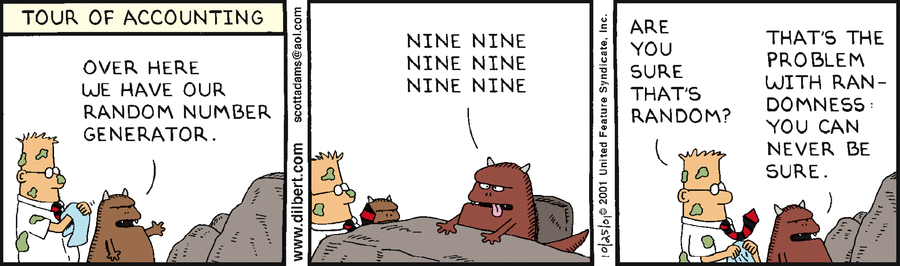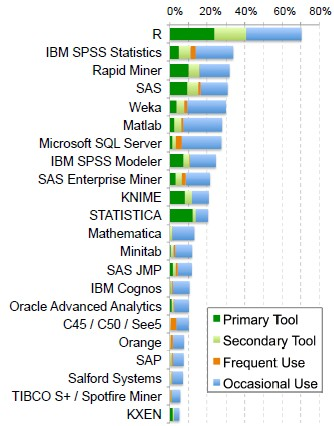# Pseudo-Randomness - Seed

The seed is the start point in the generation of pseudo-random numbers.

The random seed is any valid 32-bit integer.

Every unique seed value results in the same sequence.

Even the tiniest change in seed value will result in a radically different pseudo-random sequence and there is no way than trial and error to guess a seed value for the desired sequence.

Discover MoreNumber - Pseudo-random Numbers

Pseudo-random numbers is a sequence of numbers that is predictable if you know the seed. Because true randomness is unpredictable, this is called pseudo randomness (If you know the seed, you can predict...Number - Random (Stochastic|Independent) or (Balanced)

Think of randomness as a lack of pattern. Something random should be unpredictable. We shouldn’t be able to predict the next value of the sequence The degree to which a system has no pattern is known...R - Bootstrap

in R. Bootstrap lets you get a look at the sampling distribution of statistics, for which it's really hard to develop theoretical versions. Bootstrap gives us a really easy way of doing statistics when...R - Cluster Generation

How to generate cluster data. To generate clustered data, the mean of random generated group of data is shifted. where: the seed is set rnorm is a random generation function for the normal...R - Sample

Sample is a function that return a random subset of a data set. where:Spark - (Random) Split

randomSplit randomly splits a RDD with the provided weights. where: weights – weights for splits, will be normalized...Weka

is an open-source project in machine learning, Data Mining. is a comprehensive collection of machine-learning algorithms for data mining tasks written in Java....